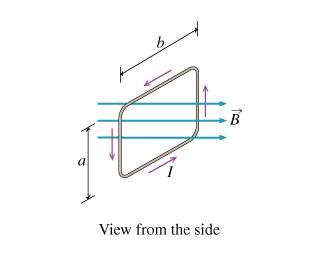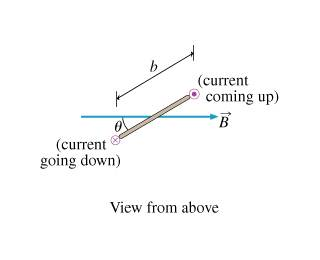# Determining the magnetic torque on a loop

• warfreak131
In summary, the conversation discussed the calculation of torque on a current loop in a uniform magnetic field, with the angle between the sides of the loop and the field being important in determining the trigonometric function to use in the equation. The angle θ represents the angle between the magnetic field and the normal to the plane of the loop, which can be visualized as a line perpendicular to the plane of the loop. The equivalent expression for torque is IABcos(θ).

## Homework Statement

A current $$I$$ flows in a plane rectangular current loop with height a and horizontal sides b. The loop is placed into a uniform magnetic field $$\vec{B}$$ in such a way that the sides of length a are perpendicular to $$\vec{B}$$ (Part A 1 figure), and there is an angle $$\theta$$ between the sides of length $$b$$ and $$\vec{B}$$ (Part A 2 figure).

Calculate $$\tau$$, the magnitude of the torque about the vertical axis of the current loop due to the interaction of the current through the loop with the magnetic field.
Express the magnitude of the torque in terms of the given variables. You will need a trigonomeric function [e.g., $$\sin(\theta)$$ or $$\cos(\theta)$$]. Use $$B$$ for the magnitude of the magnetic field.

Figure A 1Figure A 2## Homework Equations

$$\tau=IAB\sin(\theta)$$ (Assuming only 1 loop of wire)

## The Attempt at a Solution

I know that the area is $$a{\cdot}b$$, the current is $$I$$, the field is $$B$$, and the angle is $$\theta$$. I put my answer in as

$$\tau=I(a{\cdot}b)B\sin(\theta)$$

the feedback on my answer said to check my trigonometry, so I changed my answer to be the cosine instead of the sine and it was correct. Why is this? What is theta supposed to be the angle in between?

In the expression

$$\tau=IAB\sin(\theta)$$

angle θ is the angle between the magnetic field and the normal to the plane of the loop.

kuruman said:
In the expression

$$\tau=IAB\sin(\theta)$$

angle θ is the angle between the magnetic field and the normal to the plane of the loop.

so would the normal be a line perpendicular to the plane assuming its a top down view, like in figure 2?

Yes, it would be down and to the right in figure 2 as required by the right hand rule for the current shown. In short, it is the direction of the magnetic dipole moment associated with the current loop.

oh i see, so the equivalent answer would be IABsin(90-theta)) which is teh same as IABcos(theta)?

Precisely.